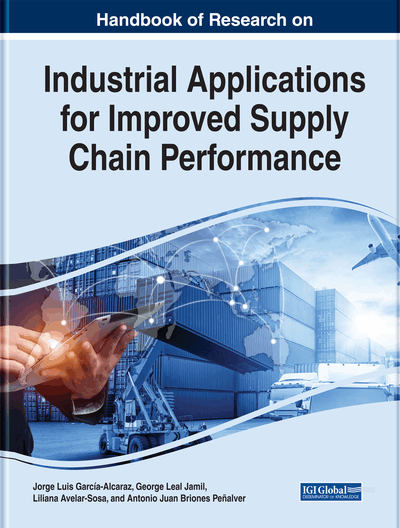# An Analysis of the Traveling Speed in the Traveling Hoist Scheduling Problem for Electroplating Processes

DOI: 10.4018/978-1-7998-0202-0.ch017
OnDemand:
(Individual Chapters)
Available
\$29.50
No Current Special Offers

## Abstract

The Hoist Scheduling Problem is combinatory, so tools such as mathematical programming need to be used to get the sequence of movements, respecting the constraints of the process by minimizing the cycle time. A sequence in which the order of movements follows the order of the process is known as the basic diagram. These schedules do not have any clearance for the hoist to make any other movements, resulting in a loss in productivity. This chapter takes the production line of a Mexican factory as a case study, analyzing the hoist's travelling speed to find sequences of movements that could improve productivity. The results of the study indicate that the cycle time has a nonlinear behavior in respect of the hoist's travelling speed and it was determined that there are travelling speeds for which sequences are obtained with enough clearance to make other movements and keep other carriers on the line. A suitable speed was estimated in the case.
Chapter Preview
Top

## Background

When the movements of the hoist are programmed, the aim is to minimize either the cycle time or to maximize the use of the crane. Because all material handling operations are developed by this mechanism, the efficiency of the production system is strongly related to the sequence of movements of the device (Che, Feng, Chen, and Chu, 2015). The HSP is an NP-hard problem. With “small” instances or with a few tanks a total enumeration can be executed to find a sequence of movement; however, the number of possible solutions grows explosively as the number of tanks in the line increases, therefore which it becomes impractical even for a computer to enumerate them all because of the time required; this is because tools such as mathematical programming are used to model the problem and we need exact methods or meta-heuristic techniques in order to find the solution (Varnier, Bachelu, & Baptiste, 1997).

According to Bloch et al. (2008) an electroplating system consists of the following elements:

• One or more input and output stations for materials.

• Containers where the pieces to be treated in the same way are placed, having been grouped in batches of a predefined size.

• A series of tanks with chemical substances.

• Hoists or robots, in charge of moving containers between tanks.

The sequence of the electroplating process establishes where each operation should be done, in what order and how long they should take; it typically consists of the following phases (Bloch et al., 2008) (Figure 1).

• Preparation (cleaning and rinsing the pieces).

• Depositing the metal by chemical or electrolytic means.

• Finishing (phosphate treatment, turning, drying, etc.)

## Key Terms in this Chapter

Electroplating Processes: Production system where the surface of a material is coated with metal in an electrochemical process.

NP-Hard Problem (Non-Deterministic-Polynomial problem): Concept of computational complexity theory to establish “how difficult it is to solve a problem using an algorithm”. The problem A is considered NP-hard when every problem B in NP can be reduced (converted) to A. The reduction allows to establish that problem A is as difficult to solve as problem B. If there is a deterministic algorithm T that solves the problem A in a time bounded by a polynomial, then the same algorithm will solve the problem B in a time bounded by a polynomial ( Dasgupta, Papadimitriou & Vazirani, 2006 ).

Cycle Time: The time that an entity spends in the system, from the beginning to the end of a process.

Mathematical Optimization: Is the selection of the best element from some set of alternatives.

Traveling Hoists: Mechanisms that are mainly used in material and load moving activities.

Time Way Diagram: Visual tool for representing the movements of the hoists between the tanks.

Scheduling: The process of arranging and controlling jobs in a machine or manufacturing process.

Mathematical Model: A description of a system using mathematical symbols.

NP: Acronym of Non-Deterministic-Polynomial Time. Concept of computational complexity theory. A class of decision problems for which a solution can be found and verified in polynomial time by special sort of algorithm; such an algorithm has the property of guessing correctly every step ( Dasgupta, Papadimitriou & Vazirani, 2006 ).

## Complete Chapter List

Search this Book:
Reset